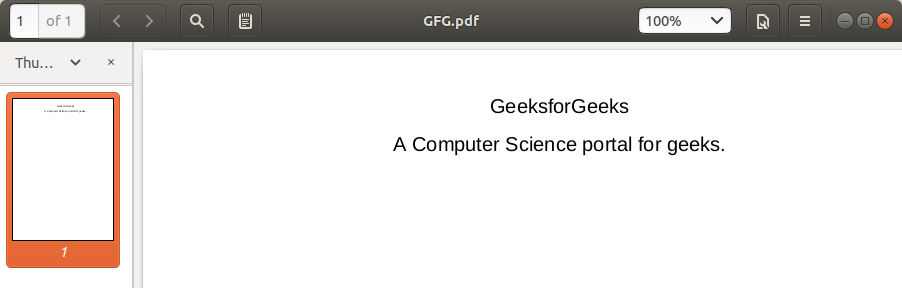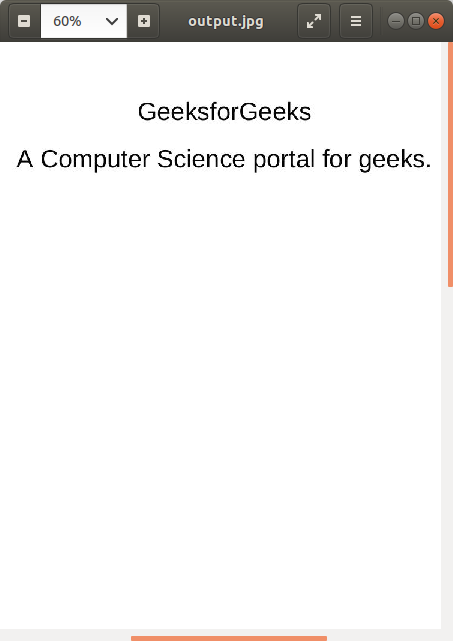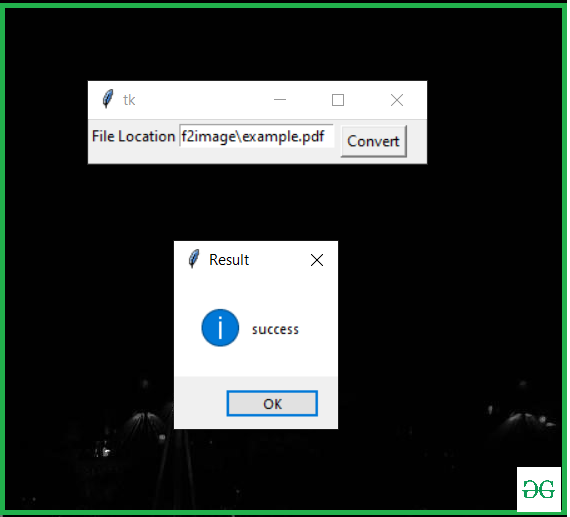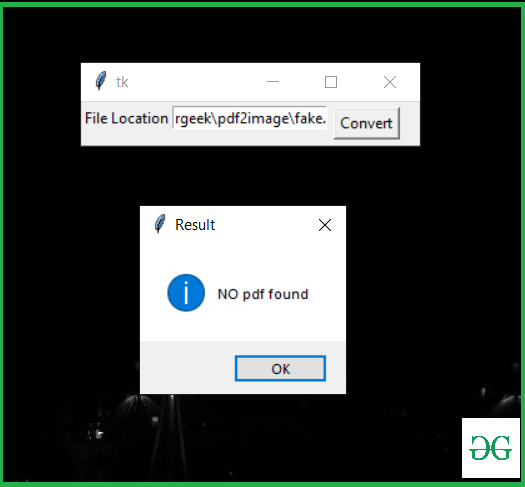# Convert PDF to Image using Python

## Convert PDF to Image using Python

Many tools are available on the internet for converting a PDF to an image. In this article, we are going to write code for converting pdf to image and make a handy application in python. Before writing the code we need to install the required module pdf2image and poppler.

### Modules Needed

• pdf2image 1.14.0: This module converts a PDF to a PIL object. To install this module type the below command in the terminal.
pip install pdf2image

You will then have to add the bin/ folder to PATH or use

poppler_path = r”C:\path\to\poppler-xx\bin” as an argument in convert_from_path.

Approach:

• Import the pdf2image module
• Store a PFD with convert_from_path()
• Save image with save()

Below is the Implementation.

PDF File used:# import module from pdf2image import convert_from_path # Store Pdf with convert_from_path function images = convert_from_path('example.pdf') for i in range(len(images)):          # Save pages as images in the pdf     images[i].save('page'+ str(i) +'.jpg', 'JPEG')

Output:Let’s write code for Application Using Tkinter: This Script implements the above Implementation into a GUI.

Below is the Implementation.

 from pdf2image import convert_from_path from tkinter import * from tkinter import messagebox def pdf2img():     try:         images = convert_from_path(str(e1.get()))         for img in images:             img.save('new_folder\output.jpg', 'JPEG')     except  :         Result = "NO pdf found"         messagebox.showinfo("Result", Result)     else:         Result = "success"         messagebox.showinfo("Result", Result) master = Tk() Label(master, text="File Location").grid(row=0, sticky=W) e1 = Entry(master) e1.grid(row=0, column=1) b = Button(master, text="Convert", command=pdf2img) b.grid(row=0, column=2,columnspan=2, rowspan=2,padx=5, pady=5)   mainloop()

Output:If there is no PDF file at your given location.Last Updated on November 13, 2021 by admin

## Python – Using 2D arrays/lists the right wayPython – Using 2D arrays/lists the right way

Python | Using 2D arrays/lists the right way Python provides many ways to create 2-dimensional

## Creating a Pandas dataframe column based on a given conditionCreating a Pandas dataframe column based on a given condition

Creating a Pandas dataframe column based on a given condition   While operating on data,

## Python – Replace multiple characters at oncePython – Replace multiple characters at once

Python | Replace multiple characters at once The replacement of one character with another is

## bool() in Pythonbool() in Python

bool() in Python Python bool() function is used to return or convert a value to a

## f-strings in Pythonf-strings in Python

f-strings in Python PEP 498 introduced a new string formatting mechanism known as Literal String Interpolation or more

## How to Get a List of Class Attributes in Python?How to Get a List of Class Attributes in Python?

How to Get a List of Class Attributes in Python? A class is a user-defined

## self in Python classself in Python class

Python Self self represents the instance of the class. By using the “self” keyword we

## Print all sublists of a list in PythonPrint all sublists of a list in Python

Print all sublists of a list in Python Given a list, print all the sublists